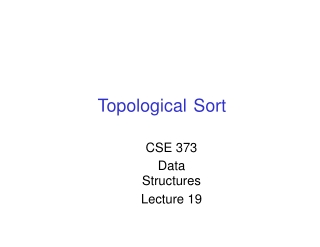DownloadDownload PresentationTopo l og ic a l

# Topo l og ic a l

Télécharger la présentation## Topo l og ic a l

- - - - - - - - - - - - - - - - - - - - - - - - - - - E N D - - - - - - - - - - - - - - - - - - - - - - - - - - -
##### Presentation Transcript

1. Topological Sort CSE 373 Data Structures Lecture 19

2. Topological Sort 142 322 143 321 Problem:Findanorderin which all these be taken. courses can 326 370 341 Example: 370 326 142 321 421 143 341 401 378 322 378 421 401 Inorder totake acourse,youmust takeallofitsprerequisitesfirst 11/27/02 TopologicalSort-Lecture19 3

3. Topological Sort Given a digraph G = (V, its vertices such that: E),findalinearorderingof for any edge (v, w) in E, v precedes win the ordering B C A F D E 11/27/02 TopologicalSort-Lecture19 4

4. Topo sort - good example B C Any linear ordering all the arrows go to inwhich A the right is avalidsolution F D E A B F C D E Note thatFcangoanywhere inthis list because itisnotconnected. 11/27/02 TopologicalSort-Lecture19 5

5. Topo sort - bad example B C Any linear ordering in which an arrow goes to the left A F is not a valid solution D E A B F C E D NO! 11/27/02 TopologicalSort-Lecture19 6

6. Not all can be Sorted • Adirectedgraphwith a cycle cannot be topologically sorted. B C A F D E 11/27/02 TopologicalSort-Lecture19 7

7. Cycles • GivenadigraphG=(V,E), acycleisa sequence of that verticesv1,v2, …,vk such › › › G k < 1 v1 = vk (vi,vi+1) in E for1<i <k. • isacyclicifithasnocycles. 11/27/02 TopologicalSort-Lecture19 8

8. Topo sortalgorithm - 1 Step1:Identifyverticesthat havenoincoming edges • The “in-degree” of these vertices iszero B C A F D E 11/27/02 Topological Sort-Lecture19 9

9. Topo sort algorithm - 1a Step1:Identifyverticesthat havenoincomingedges • • Ifnosuchvertices,graphhasonlycycle(s) (cyclic graph) Topological sort not possible–Halt. B C A Example ofacyclicgraph D 11/27/02 TopologicalSort-Lecture19 10

10. Topo sort algorithm - 1b Step1:Identifyvertices that haveno incoming edges • Select onesuch vertex Select B C A F D E 11/27/02 TopologicalSort-Lecture19 11

11. Toposort algorithm - 2 Step2: Deletethis vertexofin-degree0andall its outgoing edges output. B A from the graph. Place it in the C A F D E 11/27/02 TopologicalSort-Lecture19 12

12. Continue until done RepeatStep1 andStep2 until graph is empty Select B C A F D E 11/27/02 TopologicalSort-Lecture19 13

13. B SelectB. Copy to sorted list. Delete B and its edges. B C A B F D E 11/27/02 TopologicalSort-Lecture19 14

14. C Select C. Copy to sorted list. Delete C and its edges. C A B C F D E 11/27/02 TopologicalSort-Lecture19 15

15. D Select D. Copy to sorted list. Delete D and its edges. D A B C F D E 11/27/02 Topological Sort-Lecture19 16

16. E, F Select Select E. F. Copy Copy to to sorted sorted list. list. Delete Delete Eanditsedges. F and itsedges. D A B C E F F E 11/27/02 TopologicalSort-Lecture19 17

17. Done B C A F Removefrom algorithm and serve. D E D A B C E F 11/27/02 TopologicalSort-Lecture19 18

18. Topological Ordering Algorithm: Example v2 v3 v6 v5 v4 v7 v1 v1 Topological order:

19. Topological Ordering Algorithm: Example v2 v2 v3 v6 v5 v4 v7 Topological order: v1

20. Topological Ordering Algorithm: Example v3 v3 v6 v5 v4 v7 Topological order: v1, v2

21. Topological Ordering Algorithm: Example v6 v5 v4 v4 v7 Topological order: v1, v2, v3

22. Topological Ordering Algorithm: Example v6 v5 v5 v7 Topological order: v1, v2, v3, v4

23. Topological Ordering Algorithm: Example v6 v6 v7 Topological order: v1, v2, v3, v4, v5

24. Topological Ordering Algorithm: Example v7 v7 Topological order: v1, v2, v3, v4, v5, v6

25. Topological Ordering Algorithm: Example v2 v3 v6 v5 v4 v1 v2 v3 v4 v5 v6 v7 v7 v1 Topological order: v1, v2, v3, v4, v5, v6, v7.

26. Topological Sort Algorithm 1. 2. 3. Store each vertex’s In-Degree in an array D Initialize queue with all “in-degree=0” vertices While there are vertices remaining in the queue: (a) (b) (c) Dequeue and output a vertex Reduce In-Degree of all vertices adjacent to it by 1 Enqueue any of these vertices whose In-Degree became zero all vertices are output then success, 4. If otherwisethereis acycle. 11/27/02 TopologicalSort-Lecture19 24

27. Topological Sort Analysis • • • Initialize In-Degree array: O(|V| + |E|) Initialize Queue with In-Degree 0 vertices: O(|V|) Dequeue and output vertex: › |V| vertices, each takes only O(1) to dequeue and output: O(|V|) • Reduce In-Degree of all vertices adjacent and Enqueue any In-Degree 0 vertices: toavertex › O(|E|) • For inputgraphG=(V,E) runtime = O(|V| +|E|) › 11/27/02 Linear time! TopologicalSort-Lecture19 26